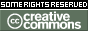REPOZYTORIUM UNIWERSYTETU
W BIAŁYMSTOKUProszę używać tego identyfikatora do cytowań lub wstaw link do tej pozycji: `http://hdl.handle.net/11320/5486`
 Tytuł: Modelling Real World Using Stochastic Processes and Filtration Autorzy: Jaeger, Peter Słowa kluczowe: stochastic process; random variablerandom variable Data wydania: 2016 Data dodania: 16-maj-2017 Wydawca: De Gruyter Open Źródło: Formalized Mathematics, Volume 24, Issue 1, pp. 1-16 Abstrakt: First we give an implementation in Mizar  basic important definitions of stochastic finance, i.e. filtration (, pp. 183 and 185), adapted stochastic process (, p. 185) and predictable stochastic process (, p. 224). Second we give some concrete formalization and verification to real world examples. In article  we started to define random variables for a similar presentation to the book . Here we continue this study. Next we define the stochastic process. For further definitions based on stochastic process we implement the definition of filtration. To get a better understanding we give a real world example and connect the statements to the theorems. Other similar examples are given in , pp. 143-159 and in , pp. 110-124. First we introduce sets which give informations referring to today (Ωnow, Def.6), tomorrow (Ωfut1 , Def.7) and the day after tomorrow (Ωfut2 , Def.8). We give an overview for some events in the σ-algebras Ωnow, Ωfut1 and Ωfut2, see theorems (22) and (23). The given events are necessary for creating our next functions. The implementations take the form of: Ωnow ⊂ Ωfut1 ⊂ Ωfut2 see theorem (24). This tells us growing informations from now to the future 1=now, 2=tomorrow, 3=the day after tomorrow. We install functions f : {1, 2, 3, 4} → ℝ as following: f1 : x → 100, ∀x ∈ dom f, see theorem (36), f2 : x → 80, for x = 1 or x = 2 and f2 : x → 120, for x = 3 or x = 4, see theorem (37), f3 : x → 60, for x = 1, f3 : x → 80, for x = 2 and f3 : x → 100, for x = 3, f3 : x → 120, for x = 4 see theorem (38). These functions are real random variable: f1 over Ωnow, f2 over Ωfut1, f3 over Ωfut2, see theorems (46), (43) and (40). We can prove that these functions can be used for giving an example for an adapted stochastic process. See theorem (49). We want to give an interpretation to these functions: suppose you have an equity A which has now (= w1) the value 100. Tomorrow A changes depending which scenario occurs − e.g. another marketing strategy. In scenario 1 (= w11) it has the value 80, in scenario 2 (= w12) it has the value 120. The day after tomorrow A changes again. In scenario 1 (= w111) it has the value 60, in scenario 2 (= w112) the value 80, in scenario 3 (= w121) the value 100 and in scenario 4 (= w122) it has the value 120. For a visualization refer to the tree: The sets w1,w11,w12,w111,w112,w121,w122 which are subsets of {1, 2, 3, 4}, see (22), tell us which market scenario occurs. The functions tell us the values to the relevant market scenario: For a better understanding of the definition of the random variable and the relation to the functions refer to , p. 20. For the proof of certain sets as σ-fields refer to , pp. 10-11 and , pp. 1-2. This article is the next step to the arbitrage opportunity. If you use for example a simple probability measure, refer, for example to literature , pp. 28-34, , p. 6 and p. 232 you can calculate whether an arbitrage exists or not. Note, that the example given in literature  needs 8 instead of 4 informations as in our model. If we want to code the first 3 given time points into our model we would have the following graph, see theorems (47), (44) and (41): The function for the “Call-Option” is given in literature , p. 28. The function is realized in Def.5. As a background, more examples for using the definition of filtration are given in , pp. 185-188. Afiliacja: Jaeger Peter - Siegmund-Schacky-Str. 18a 80993 Munich, Germany URI: http://hdl.handle.net/11320/5486 DOI: 10.1515/forma-2016-0001 ISSN: 1426-26301898-9934 Typ Dokumentu: Article Występuje w kolekcji(ach): Formalized Mathematics, 2016, Volume 24, Issue 1

Pliki w tej pozycji:
Plik Opis RozmiarFormat
Pozycja ta dostępna jest na podstawie licencji Licencja Creative Commons CCL